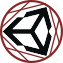#Uninomicon

Documenting the dark corners of the Unity Engine.

physicmaterial

# PhysicMaterial

## Friction values

Physic Material 1) is a small data object that can be attached to Colliders to provide parameters for their collision behavior.

If NVidia PhysX is being used 2), static and dynamic friction values operate according to the Coulomb model of friction. For a Rigidbody sliding horizontally across another collider, the “friction force” points in the opposite direction of the Rigidbody's momentum and its magnitude is:

`N * µ`

where:

• N is the normal force, i.e. mass * gravity
• µ is (dynamic or static) friction coefficient 3)

Loosely put: “friction” counteracts a Rigidbody's momentum with a minimum magnitude of 0 and a maximum of m*g. The friction values are clamped 0-1 because values > 1 would “create” energy.

## Friction combine

The value µ from the friction calculation above is calculated from both colliding materials. The “friction combine” field determines what calculation is used. If two colliding Physic Materials have different “friction combine” values, the Unity documentation correctly states that the order of priority goes Maximum > Multiply > Minimum > Average. However, this does not respect the underlying int values of the PhysicMaterialCombine:

• 0 Average
• 1 Multiply
• 2 Minimum
• 3 Maximum

This will trip you up if you expect these to match.

1)
Note the incorrect spelling!
2)
Currently this is the default physics system
3)
See “Friction Combine”